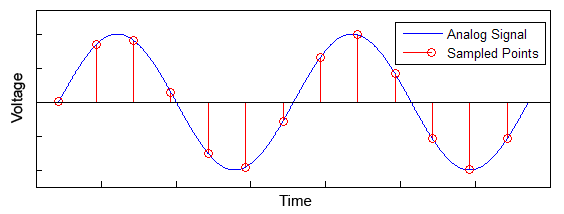# Determination of the frequency of a sampled signal

In summary, to accurately determine the received frequency, the receiver must synchronize its local oscillator to the carrier phase and frequency. Once synchronized, the receiver uses cross-correlation to determine which symbol has the highest correlation.f

Hello everyone,

I have a question regarding data reconstruction over RF, especially in the case of FM. Let us say I have a trivial case where I have a carrier frequency of fo and a bandwidth of fbw. I want to setup a scheme such that fo + fbw is a 1 and fo - fbw is a 0. (I'm naively under the impression that this is, in general, how FM works...)

At the receiver side, the hardware is measuring (and sampling) voltage over time. If the samples are being taken at higher than the Nyquist frequency, I should (in theory) be able to reconstruct the original signal. But how does one calculate the frequency received in real time?

In other words, at what point does one know "Ah this is at fo + fbw, therefore a 1" during the sampling process? Does it take multiple samples, or is there some way to immediately calculate this, and with what level of confidence?

An example image: If I'm sampling a signal (red circles), where can I confidently conclude that the frequency is either fo + fbw or fo - fbw?Thanks!

#### Attachments

Last edited by a moderator:
For frequency modulation, with a binary state (either +Fbw or -Fbw) you should see it sometimes faster, and sometimes slower. Are you indicating that you know nothing about what f0 carrier frequency the person is transmitting at? Before a signal is sampled, it ahouldhave first been passed through some analog filters. Like if you tune your radio to 99.1 MHz, it has gone through a bandpass filter first.

Yes, exactly, sometimes the freq is higher or lower, but how quickly can your hardware determine that? In my ignorance, I feel you'd have to sample some stretch, like maybe 2 or more periods, before knowing the frequency with high confidence.

This seems an important feature because complicated protocols like QAM require real-time sampling and reconstruction of data. I'm trying to figure it out on a really basic level first...

• The modulation you describe is called 2-FSK or BFSK (frequency shift keying with 2 symbols, or binary FSK).
• The receiver synchronizes its local oscillator to the carrier phase and frequency (look up Costasop, for instance) so that the RF is downconverted to a known and stable low frequency. That frequency is typically zero so the two symbols are +f_BW and -f_BW.
• Each symbol is a predefined number of cycles. The longer the symbol, the more robust the system is to noise and interference, at the expense of a lower data rate.
• Detection is often performed by a matched filter. The sampled waveform is cross-correlated against a stored reference copy of each symbol (frequency), and 1 or 0 is declared depending on which has the highest correlation.
• An alternate approach is to take an FFT (assuming the number of samples is even). An integer number of cycles per symbol is often used in this case.
• There are many versions of FSK, with memory to give one example, that give certain advantages at the cost of greater complexity. You can search FSK for more info.

•• The modulation you describe is called 2-FSK or BFSK (frequency shift keying with 2 symbols, or binary FSK).
• The receiver synchronizes its local oscillator to the carrier phase and frequency (look up Costasop, for instance) so that the RF is downconverted to a known and stable low frequency. That frequency is typically zero so the two symbols are +f_BW and -f_BW.
• Each symbol is a predefined number of cycles. The longer the symbol, the more robust the system is to noise and interference, at the expense of a lower data rate.
• Detection is often performed by a matched filter. The sampled waveform is cross-correlated against a stored reference copy of each symbol (frequency), and 1 or 0 is declared depending on which has the highest correlation.
• An alternate approach is to take an FFT (assuming the number of samples is even). An integer number of cycles per symbol is often used in this case.
• There are many versions of FSK, with memory to give one example, that give certain advantages at the cost of greater complexity. You can search FSK for more info.

Awesome, thank you for the detailed explanation. I can follow the logic of BPSK, QAM, etc, including error correction and other logic techniques. It was the part where the value was determined to be at +f_BW or -f_BW that was alluding me (or determined to be at some IQ value, etc). Your statement about each symbol having a pre-determined number of cycles helps a lot, and it also helps clarify what I've read about needing to be "locked" between Tx and Rx (otherwise your symbols are incorrect).

LOL, I just saw the emoji that I managed to insert in place of "Loop." So much for typing on a little phone screen!